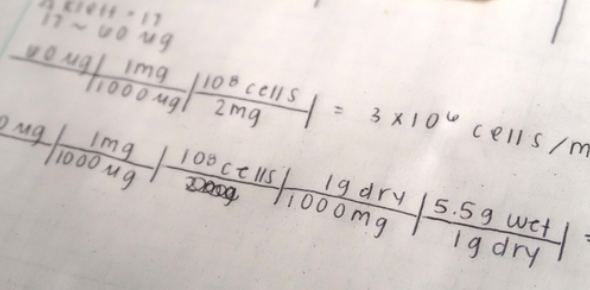# Factor-label method Quiz

Approved & Edited by ProProfs Editorial Team
The editorial team at ProProfs Quizzes consists of a select group of subject experts, trivia writers, and quiz masters who have authored over 10,000 quizzes taken by more than 100 million users. This team includes our in-house seasoned quiz moderators and subject matter experts. Our editorial experts, spread across the world, are rigorously trained using our comprehensive guidelines to ensure that you receive the highest quality quizzes.
| By Keith Foster
Keith Foster, Content Moderator
Keith is a seasoned quiz creator with a wealth of experience in crafting engaging and informative quizzes, including notable expertise in personality quizzes that captivate diverse audiences. His passion for quizzing and knack for delivering enjoyable quiz experiences make him a valuable asset to the world of quiz creation at ProProfs.com. Keith loves crafting compelling personality quizzes on various topics.
Quizzes Created: 1157 | Total Attempts: 1,506,798
Questions: 10 | Attempts: 493SettingsFor students of math, we have brought a simple Factor-label method quiz right here. What do you know about this factor-label method? Do you know the use of it? The factor-label method is also known as dimensional analysis or unit conversions. This method is used to convert from one unit of measurement to another unit. Here, we will see how skilled you are with the factor-label method. Answer some questions, score high, and prove your knowledge.

• 1.

### If 1.000 kg is 2.205 pounds, 8.000 kg is

• A.

17.637 pounds

• B.

19.64 pounds

• C.

15.64 pounds

• D.

10.65 pounds

A. 17.637 pounds
Explanation
The correct answer can be determined by multiplying the given weight in kilograms (8.000 kg) by the conversion factor of 2.205 pounds per 1 kilogram. This results in 17.64 pounds, which is closest to the answer provided (17.637 pounds).

Rate this question:

• 2.

### If 1 pound is 0.45 kg, how many pounds is 0.9 kg?

• A.

0.5

• B.

1.5

• C.

2

• D.

3

C. 2
Explanation
To find out how many pounds is 0.9 kg, we can use the conversion factor given in the question, which is 1 pound is equal to 0.45 kg. By dividing 0.9 kg by 0.45 kg/pound, we can cancel out the units and find that 0.9 kg is equal to 2 pounds. Therefore, the correct answer is 2.

Rate this question:

• 3.

### How many yards are 15 feet?

• A.

5

• B.

7

• C.

11

• D.

15

A. 5
Explanation
There are 3 feet in 1 yard. Therefore, to convert 15 feet to yards, we divide 15 by 3. This gives us 5, which is the correct answer.

Rate this question:

• 4.

### Convert 400 kgs into quintals.

• A.

1.5 quintals

• B.

2 quintals

• C.

3 quintals

• D.

4 quintals

D. 4 quintals
Explanation
To convert kilograms into quintals, we divide the given weight by 100. In this case, 400 kgs divided by 100 equals 4 quintals.

Rate this question:

• 5.

### 7 inches are equal to

• A.

0.19 yards

• B.

0.23 yards

• C.

0.53 yards

• D.

1 yard

A. 0.19 yards
Explanation
To convert inches to yards, divide the number of inches by 36. In this case, 7 inches divided by 36 equals approximately 0.19 yards.

Rate this question:

• 6.

### How many inches are two yards?

• A.

32

• B.

56

• C.

72

• D.

77

C. 72
Explanation
Two yards is equal to 72 inches. This is because there are 36 inches in one yard, so when you double that to get two yards, you get 72 inches.

Rate this question:

• 7.

### 5 centimeters are equal to

• A.

1.96 inches

• B.

1.99 inches

• C.

2.96 inches

• D.

1.99 inches

A. 1.96 inches
Explanation
5 centimeters are equal to 1.96 inches. This conversion is based on the fact that 1 inch is equal to 2.54 centimeters. Therefore, to convert centimeters to inches, we divide the length in centimeters by 2.54. In this case, 5 centimeters divided by 2.54 equals 1.96 inches.

Rate this question:

• 8.

### How many tons are 3500 kgs?

• A.

2.5 tons

• B.

3 tons

• C.

3.5 tons

• D.

4 tons

C. 3.5 tons
Explanation
3500 kilograms is equal to 3.5 tons. This is because 1 ton is equal to 1000 kilograms. Therefore, to convert kilograms to tons, you divide the number of kilograms by 1000. In this case, 3500 divided by 1000 equals 3.5.

Rate this question:

• 9.

### How many yards are 2 meters?

• A.

1.98

• B.

2.18

• C.

2.36

• D.

3

B. 2.18
Explanation
The conversion factor for meters to yards is 1.0936. To convert 2 meters to yards, we multiply 2 by 1.0936. The result is 2.1872, which can be rounded to 2.18. Therefore, 2 meters is equal to 2.18 yards.

Rate this question:

• 10.

### If 1 foot is equal to 12 inches, then how many feet will be equal to 42 inches?

• A.

2.2 feet

• B.

2.5 feet

• C.

3 feet

• D.

3.5 feet

D. 3.5 feet
Explanation
Since 1 foot is equal to 12 inches, to find how many feet are equal to 42 inches, we divide 42 by 12. This gives us 3.5, so the answer is 3.5 feet.

Rate this question:

Related TopicsBack to top